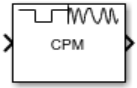# CPM Modulator Baseband

Modulate signal using CPM method

• Library:
• Communications Toolbox / Modulation / Digital Baseband Modulation / CPM

•## Description

The CPM Modulator Baseband block modulates an input signal using the continuous phase modulation (CPM) method. The output of the modulator is a baseband representation of the modulated signal.

For more information about the modulation and the filtering applied, see CPM Modulation and Pulse Shape Filtering.

## Ports

### Input

expand all

Input signal, specified as a scalar or column vector.

When the Input type parameter is set to `Integer`, the block accepts odd integers in the range [ –(M–1), (M–1)]. M is the modulation order which is specified by the M-ary number parameter.

When the Input type parameter is set to `Bit`, the block accepts binary-valued inputs that represent integers. The block collects binary-valued signals into groups of k = log2(M) bits. k is the number of bits per symbol and M is the modulation order. The input vector length must be an integer multiple of k. The block maps each group of k bits onto a symbol, as specified by the Symbol set ordering parameter. For each group of k bits, the block outputs one modulated symbol, oversampled by the Samples per symbol parameter value.

#### Supported Data Types

• Double-precision floating point

• Boolean is permitted when Input type is set to `Bit`

• 8-, 16-, and 32-bit signed integers are permitted when Input type is set to `Integer`

Data Types: `double` | `Boolean` | `int8` | `int16` | `int32`

### Output

expand all

Output signal, returned as a scalar or column vector.

• When the Input type parameter is set to `Integer`, the block outputs one modulated symbol for each input symbol.

• When the Input type parameter is set to `Bit`, the block outputs one modulated symbol for each group of k bits.

In both cases, the modulated symbols are oversampled by the Samples per symbol parameter value.

Data Types: `double` | `single`

For more information on the processing rates, see Single-Rate Processing, and Multirate Processing.

## Parameters

expand all

Modulation order, specified as a power-of-two scalar. The modulation order, M = 2k specifies the number of points in the signal constellation, where k is a positive integer indicating the number of bits per symbol.

Indicates whether the input consists of integers or groups of bits, specified as `Integer` or `Bit`.

Symbol mapping of bit inputs, specified as `Binary` or `Gray`.

• Set this parameter to `Binary` to map symbols using natural binary-coded ordering.

• Set this parameter to `Gray` to map symbols using Gray-coded ordering.

#### Dependencies

To enable this parameter, set Input type to `Bit`.

Modulation index {hi}, specified as a nonnegative scalar or column vector.

{h} represents a sequence of modulation indices. For more information, see CPM Modulation.

Type of pulse shaping used to smooth the phase transitions of the modulated signal, specified as `Rectangular`, `Raised Cosine`, ```Spectral Raised Cosine```, `Gaussian`, or `Tamed FM`. For more information on the filtering options, see Pulse Shape Filtering.

Main lobe duration of the largest lobe in the spectral raised cosine pulse, specified as a positive integer representing the number of symbol intervals used by the modulator to pulse-shape the modulated signal.

#### Dependencies

To enable this parameter, set Frequency pulse shape to ```Spectral Raised Cosine```.

Rolloff factor of the spectral raised cosine pulse, specified as a scalar from 0 to 1.

#### Dependencies

To enable this parameter, set Frequency pulse shape to ```Spectral Raised Cosine```.

Product of the bandwidth and symbol time of the Gaussian pulse shape, specified as a positive scalar. Use BT product to reduce the bandwidth, at the expense of increased intersymbol interference.

#### Dependencies

To enable this parameter, set Frequency pulse shape to `Gaussian`.

Length of the frequency pulse shape in symbol intervals, specified as a positive integer. For more information on the frequency pulse length, refer to LT in Pulse Shape Filtering.

Symbol prehistory, specified as scalar or vector with odd integer elements in the range [– (M-ary number – 1), (M-ary number – 1)]. This parameter defines the data symbols used by the modulator prior to the first call of the block in reverse chronological order. When you specify this parameter as a vector, the length must be (Pulse length (symbol intervals) – 1).

Initial phase offset in radians of the modulated waveform, specified as a scalar.

Symbol sampling rate, specified as a positive scalar. This parameter represents the number of samples output for each integer or binary word input. For all nonbinary schemes, as defined by the pulse shapes, this value must be greater than 1.

Block processing rate, specified as one of these options:

• `Enforce single-rate processing` — The input and output signals have the same sample time. The block implements the rate change by making a size change at the output when compared to the input. The output width equals the product of the number of symbols and the Samples per symbol parameter value.

• `Allow multirate processing` — The input and output signals have different sample times. The output sample time equals the symbol period divided by the Samples per symbol parameter value.

Output data type, specified as `double` or `single`.

## Block Characteristics

 Data Types `Boolean` | `double` | `integer` | `single` Multidimensional Signals `no` Variable-Size Signals `no`